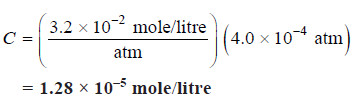# Henry’s law – a form of distribution law

## Henry’s law statement

– Henry’s law states:

at a constant temperature the solubility of a gas in a liquid is proportional to the pressure of the gas above it.

– Henry’s law may be mathematically expressed as

C = kP

where:

C is the solubility (or concentration),

k is a constant

P is the pressure of the gas,

k is called Henry’s constant.

## Explanation of Henry’s law

– Henry’s law is, in fact, a form of Distribution law.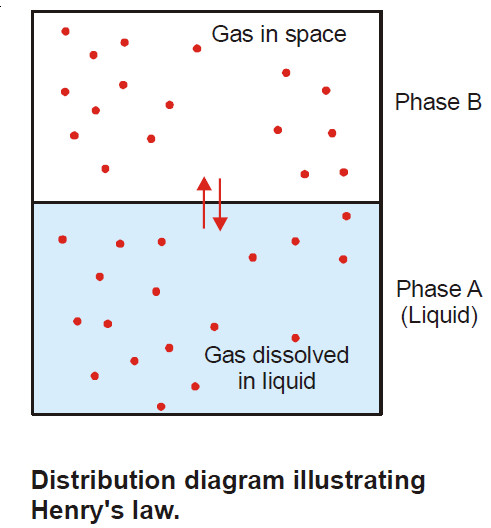– If a vessel containing a liquid and a gas is shaken, at equilibrium the gas can be regarded as distributed between the liquid (Phase A) and the space above (Phase B).

– The influence of partial pressure on solubility is utilized in making carbonated beverages like beer, champagne, and many soft drinks.

– So called ‘soda water’ is bottled under a carbon dioxide pressure of about 4 atm.

– When the bottle is opened to the air, the partial pressure of CO2 above the solution is decreased (about 0.001 atm), and CO2 bubbles out.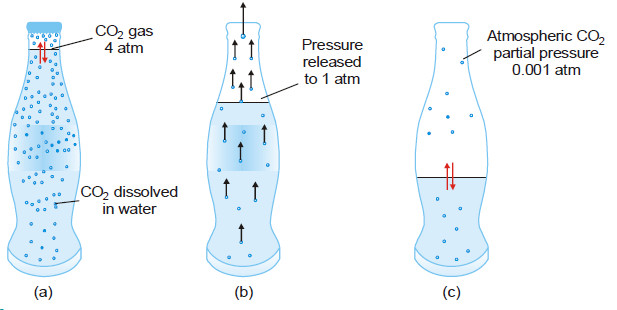(a) CO gas at 4 atm in equilibrium with dissolved CO2 resulting in high solubility of CO2 ;

(b) In opened bottle pressure is released to 1 atm and hence equilibrium shifted upward, gas bubbles evolved causing brisk effervescence;

(c) Partial pressure of CO2 in air being 0.001 atm, practically the whole of CO2 is removed from solution, leaving the soft drink flat as the equilibrium is established.

## Henry’s Law equation

Let:

C1 be the concentration of the gas in phase B

C be the concentration of the gas in phase A

Applying the Distribution law: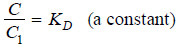We know that molar concentration of gas is proportional to its pressure, P.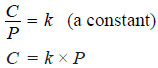This is Henry’s Law equation.

– Like distribution law, Henry’s law holds good for dilute solutions of gases which do not react with the solvent.

– If a mixture of gases is in contact with a liquid, the partial pressure of an individual gas, not their total pressure, determines the mass of each gas dissolving.

– In such a case, the solubility of each gas is proportional to its partial pressure.

## Solved problems

Solved problem(1): The solubility of pure oxygen in water at 20º C and 1.00 atm pressure is 1.38 × 10–3 mole/litre. Calculate the concentration of O2 (mole/litre) at 20ºC and a partial pressure of 0.21 atm.

Solution:

Calculation of Henry’s Constant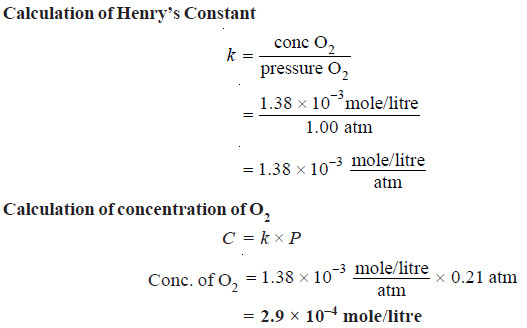Solved problem(1): A soda-water bottle was opened and the soft drink allowed to come to equilibrium at 25ºC with air containing carbon dioxide at a pressure of 4.0 × 10–4 atm. Find the concentration of CO2 in the soda after it had stood open and come to equilibrium. The Henry’s constant for aqueous solution of CO2 at 25º is: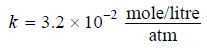Solution:

C = kP       (Henry’s law)

Substituting into Henry’s law equation, we get for the opened soft drink at equilibrium with atmospheric CO2,